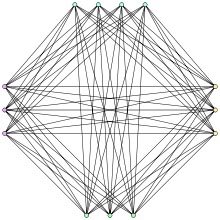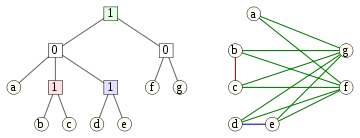# Cograph

﻿
CographThe Turán graph T(13,4), an example of a cograph

In graph theory, a cograph, or complement-reducible graph, or P4-free graph, is a graph that can be generated from the single-vertex graph K1 by complementation and disjoint union. That is, the family of cographs is the smallest class of graphs that includes K1 and is closed under complementation and disjoint union.

Cographs have been discovered independently by several authors since the 1970s; early references include Jung (1978), Lerchs (1971), Seinsche (1974), and Sumner (1974). They have also been called D*-graphs (Jung 1978), hereditary Dacey graphs (Sumner 1974), and 2-parity graphs (Burlet & Uhry 1984).

See, e.g., Brandstädt, Le & Spinrad (1999) for more detailed coverage of cographs, including the facts presented here.

## Definition and characterization

Any cograph may be constructed using the following rules:

1. any single vertex graph is a cograph;
2. if G is a cograph, so is its complement$\overline{G}$;
3. if G and H are cographs, so is their disjoint union$G\cup H$.

Several alternative characterizations of cographs can be given. Among them:

• A cograph is a graph which does not contain the path P4 on 4 vertices (and hence of length 3) as an induced subgraph. That is, a graph is a cograph if and only if for any four vertices v1,v2,v3,v4, if {v1,v2},{v2,v3} and {v3,v4} are edges of the graph then at least one of {v1,v3},{v1,v4} or {v2,v4} is also an edge (Corneil, Lerchs & Burlingham 1981).
• A cograph is a graph all of whose induced subgraphs have the property that any maximal clique intersects any maximal independent set in a single vertex.
• A cograph is a graph in which every nontrivial induced subgraph has at least two vertices with the same neighbourhoods.
• A cograph is a graph in which every connected induced subgraph has a disconnected complement.
• A cograph is a graph all of whose connected induced subgraphs have diameter at most 2.
• A cograph is a graph in which every connected component is a distance-hereditary graph with diameter at most 2.
• A cograph is a graph with clique-width at most 2 (Courcelle & Olariu 2000).

All complete graphs, complete bipartite graphs, threshold graphs, and Turán graphs are cographs. Every cograph is distance-hereditary, a comparability graph, and perfect.

Cographs are the comparability graphs of series-parallel partial orders (Jung 1978).

## CotreesA cotree and the corresponding cograph. Each edge (u,v) in the cograph has a matching color to the least common ancestor of u and v in the cotree.

A cotree is a tree in which the internal nodes are labeled with the numbers 0 and 1. Every cotree T defines a cograph G having the leaves of T as vertices, and in which the subtree rooted at each node of T corresponds to the induced subgraph in G defined by the set of leaves descending from that node:

• A subtree consisting of a single leaf node corresponds to an induced subgraph with a single vertex.
• A subtree rooted at a node labeled 0 corresponds to the union of the subgraphs defined by the children of that node.
• A subtree rooted at a node labeled 1 corresponds to the join of the subgraphs defined by the children of that node; that is, we form the union and add an edge between every two vertices corresponding to leaves in different subtrees. Alternatively, the join of a set of graphs can be viewed as formed by complementing each graph, forming the union of the complements, and then complementing the resulting union.

An equivalent way of describing the cograph formed from a cotree is that two vertices are connected by an edge if and only if the lowest common ancestor of the corresponding leaves is labeled by 1. Conversely, every cograph can be represented in this way by a cotree. If we require the labels on any root-leaf path of this tree to alternate between 0 and 1, this representation is unique (Corneil, Lerchs & Burlingham 1981).

## Computational properties

Cographs may be recognized in linear time, and a cotree representation constructed, using modular decomposition (Corneil, Perl & Stewart 1985), partition refinement (Habib & Paul 2005), or split decomposition (Gioan & Paul 2008). Once a cotree representation has been constructed, many familiar graph problems may be solved via simple bottom-up calculations on the cotrees.

For instance, to find the maximum clique in a cograph, compute in bottom-up order the maximum clique in each subgraph represented by a subtree of the cotree. For a node labeled 0, the maximum clique is the maximum among the cliques computed for that node's children. For a node labeled 1, the maximum clique is the union of the cliques computed for that node's children, and has size equal to the sum of the children's clique sizes. Thus, by alternately maximizing and summing values stored at each node of the cotree, we may compute the maximum clique size, and by alternately maximizing and taking unions, we may construct the maximum clique itself. Similar bottom-up tree computations allow the maximum independent set, vertex coloring number, maximum clique cover, and Hamiltonicity (that is the existence of a Hamiltonian cycle) to be computed in linear time from a cotree representation of a cograph. One can also use cotrees to determine in linear time whether two cographs are isomorphic.

Wikimedia Foundation. 2010.

### Look at other dictionaries:

• cograph — noun A graph formed from another by complementation and disjoin union …   Wiktionary

• Quasi-threshold graph — In graph theoretic mathematics, a quasi threshold graph is a graph that can be constructed using the following rules: # K 1 is a quasi threshold graph #If G is a quasi threshold graph, then so is the graph obtained by adding a new vertex… …   Wikipedia

• Co-Graph — In der Informatik ist ein Co Graph ein ungerichteter Graph    , welcher sich mit bestimmten elementaren Operationen konstruieren lässt. Auf Co Graphen lassen sich viele schwere Probleme wie z. B. UNABHÄNGIGE MENGE, CLIQUE und… …   Deutsch Wikipedia

• Complement graph — The Petersen graph (on the left) and its complement graph (on the right). In graph theory, the complement or inverse of a graph G is a graph H on the same vertices such that two vertices of H are adjacent if and only if they are not adjacent in G …   Wikipedia

• Threshold graph — In graph theory, a threshold graph is a graph that can be constructed from a one vertex graph by repeated applications of the following two operations: #Addition of a single isolated vertex to the graph #Addition of a single dominating vertex to… …   Wikipedia

• Turán graph — The Turán graph T(13,4) Named after Pál Turán v · …   Wikipedia

• Clique (graph theory) — A graph with 23 1 vertex cliques (its vertices), 42 2 vertex cliques (its edges), 19 3 vertex cliques (the light blue triangles), and 2 4 vertex cliques (dark blue). Six of the edges and 11 of the triangles form maximal cliques. The two dark blue …   Wikipedia

• List of mathematics articles (C) — NOTOC C C closed subgroup C minimal theory C normal subgroup C number C semiring C space C symmetry C* algebra C0 semigroup CA group Cabal (set theory) Cabibbo Kobayashi Maskawa matrix Cabinet projection Cable knot Cabri Geometry Cabtaxi number… …   Wikipedia

• Modular decomposition — In graph theory, the modular decomposition is a decomposition of an undirected graph into subsets of vertices called modules. A module is a generalization of a connected component of a graph. Unlike connected components, however, one module can… …   Wikipedia

• Split graph — In graph theory, a split graph is a graph in which the vertices can be partitioned into a clique and an independent set. Split graphs were first studied by Földes and Hammer in two papers in 1977, and independently introduced by Tyshkevich and… …   Wikipedia